# Derivation of PV=nRT, The Equation of Ideal Gas

According to the kinetic theory of gas,

– Gases are composed of very small molecules and their number of molecules is very large.
– These molecules are elastic.
– They are negligible size compare to their container.
– Their thermal motions are random.

To begin, let’s visualize a rectangular box with length L, areas of ends A1 and A2. There is a single molecule with speed vx traveling left and right to the end of the box by colliding with the end walls.

The time between collisions with the wall is the distance of travel between wall collisions divided by the speed.

1.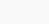The frequency of collisions with the wall in collisions per second is

2.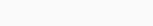According to Newton, force is the time rate of change of the momentum

3.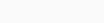The momentum change is equal to the momentum after collision minus the momentum before collision. Since we consider the momentum after collision to be mv, the momentum before collision should be in opposite direction and therefore equal to –mv.

4.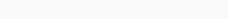According to equation #3, force is the change in momentum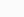divided by change in time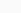.  To get an equation of average force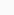in term of particle velocity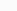, we take change in momemtummultiply by the frequency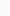from equation #2.

5.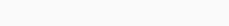The pressure, P, exerted by a single molecule is the average force per unit area, A. Also V=AL which is the volume of the rectangular box.

6.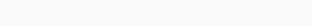Let’s say that we have N molecules of gas traveling on the x-axis. The pressure will be

7.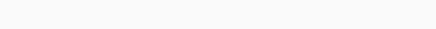To simplify the situation we will take the mean square speed of N number of molecules instead of summing up individual molecules. Therefore, equation #7 will become

8.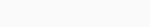Earlier we are trying to simplify the situation by only considering that a molecule with mass m is traveling on the x axis.  However, the real world is much more complicated than that. To make a more accurate derivation we need to account all 3 possible components of the particle’s speed, vx, vy and vz.

9.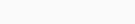Since there are a large number of molecules we can assume that there are equal numbers of molecules moving in each of co-ordinate directions.

10.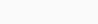Because the molecules are free too move in three dimensions, they will hit the walls in one of the three dimensions one third as often. Our final pressure equation becomes

11.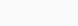However to simplify the equation further, we define the temperature, T, as a measure of thermal motion of gas particles because temperature is much easier to measure than the speed of the particle. The only energy involve in this model is kinetic energy and this kinetic enery is proportional to the temperature T.

12.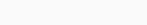To combine the equation #11 and #12 we solve kinetic energy equation #12 for mv2

13.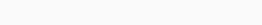Since the temperature can be obtained easily with simple daily measurement like a thermometer, we will now replace the result of kinetic equation #13 with with a constant R times the temperature, T. Again, since T is proportional to the kinentic energy it is logical to say that T times k is equal to the kinetic energy E. k, however, will currently remains unknown.

14.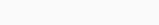Combining equation #14 with #11, we get:

15.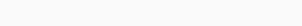Because a molecule is too small and therefore impractical we will take the number of molecules, N and divide it by the Avogadro’s number, NA= 6.0221 x 1023/mol to get n (the number of moles)

16.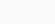Since N is divided by Na, k must be multiply by Na to preserve the original equation. Therefore, the constant R is created.

17.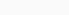Now we can achieve the final equation by replacing N (number of melecules) with n (number of moles) and k with R.

17.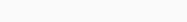Calculation of R & k

According to numerous tests and observations, one mole of gas is a 22.4 liter vessel at 273K exerts a pressure of 1.00 atmosphere (atm). From the ideal gas equation above:

A.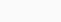B.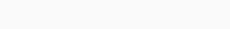C.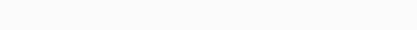### 15 responses to “Derivation of PV=nRT, The Equation of Ideal Gas”

1.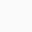harshil says:

Best explanation i have ever read…..!!!!!

2.John Thompson says:

Thanks for posting this.

The model does not account for directions other than x, y and z, that is at various angles to the planes of the box. I assume that the model boils down to the results of a more rigorous vectorial analysis, but I cannot see it intuitively.

3.bharath says:

simply tremendous

4.DEEPAK POONIA says:

a very nice explanation…………

5.Gideon says:

thanks a lot

6.kaustav says:

plz give me the diduction of p=1/3 rou c^2 (c=rms velocity) accoding to maxwell spee distribution law

7.de masakhwi says:

A nice explanation. But I think there is assumption that the molecules move in the th eeraxes :cxy,z. We need to make some adjustments.

8.Tharu says:

wow…really nice..thanks alot

9.raj says:

good explanation

10.Zadock masakhwi says:

the equation is quite clear and correct to the point. however it should be known to the reader that assumptions are made that
1. the molecules of the gas occupy negligible volume.
2. the pressure due to attraction between the molecules is negligible.
The solutions to gases using this formula and when the correction term is used are relatively similar. there is only an error of atmost 4% with most calculations. This a nice derivation!

11.aishwarya says:

it was very helpful for me

12.DonaldR says:

Splendid explanation. Thanks.

13.Munir Ahmad says:

best explanation

14.PRINCE ERYQ says:

Best explanation ever seen thanx now i can understand more

15.anu farzana says: